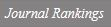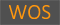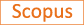A Lagrangian–DNN relaxation: a fast method for computing tight lower bounds for a class of quadratic optimization problems

Title
A Lagrangian–DNN relaxation: a fast method for computing tight lower bounds for a class of quadratic optimization problems
Authors
Kim S.Kojima M.Toh K.-C.
Ewha Authors
SCOPUS Author ID
김선영Issue Date
2016
Journal Title
Mathematical Programming
ISSN
0025-5610Citation
Mathematical Programming vol. 156, no. 42371, pp. 161 - 187
Keywords
Bisection methodIterative solverLinearly constrained quadratic optimization problems with complementarity constraintsThe Lagrangian–conic relaxation
Publisher
Springer Verlag
Indexed
SCI; SCIE; SCOPUSDocument Type
Article
Abstract
We propose an efficient computational method for linearly constrained quadratic optimization problems (QOPs) with complementarity constraints based on their Lagrangian and doubly nonnegative (DNN) relaxation and first-order algorithms. The simplified Lagrangian–completely positive programming (CPP) relaxation of such QOPs proposed by Arima, Kim, and Kojima in 2012 takes one of the simplest forms, an unconstrained conic linear optimization problem with a single Lagrangian parameter in a CPP matrix variable with its upper-left element fixed to 1. Replacing the CPP matrix variable by a DNN matrix variable, we derive the Lagrangian–DNN relaxation, and establish the equivalence between the optimal value of the DNN relaxation of the original QOP and that of the Lagrangian–DNN relaxation. We then propose an efficient numerical method for the Lagrangian–DNN relaxation using a bisection method combined with the proximal alternating direction multiplier and the accelerated proximal gradient methods. Numerical results on binary QOPs, quadratic multiple knapsack problems, maximum stable set problems, and quadratic assignment problems illustrate the superior performance of the proposed method for attaining tight lower bounds in shorter computational time. © 2015, Springer-Verlag Berlin Heidelberg and Mathematical Optimization Society.
DOI
10.1007/s10107-015-0874-5
Appears in Collections:
자연과학대학 > 수학전공 > Journal papers
Files in This Item:
There are no files associated with this item.
Export
RIS (EndNote)
XLS (Excel)
XML